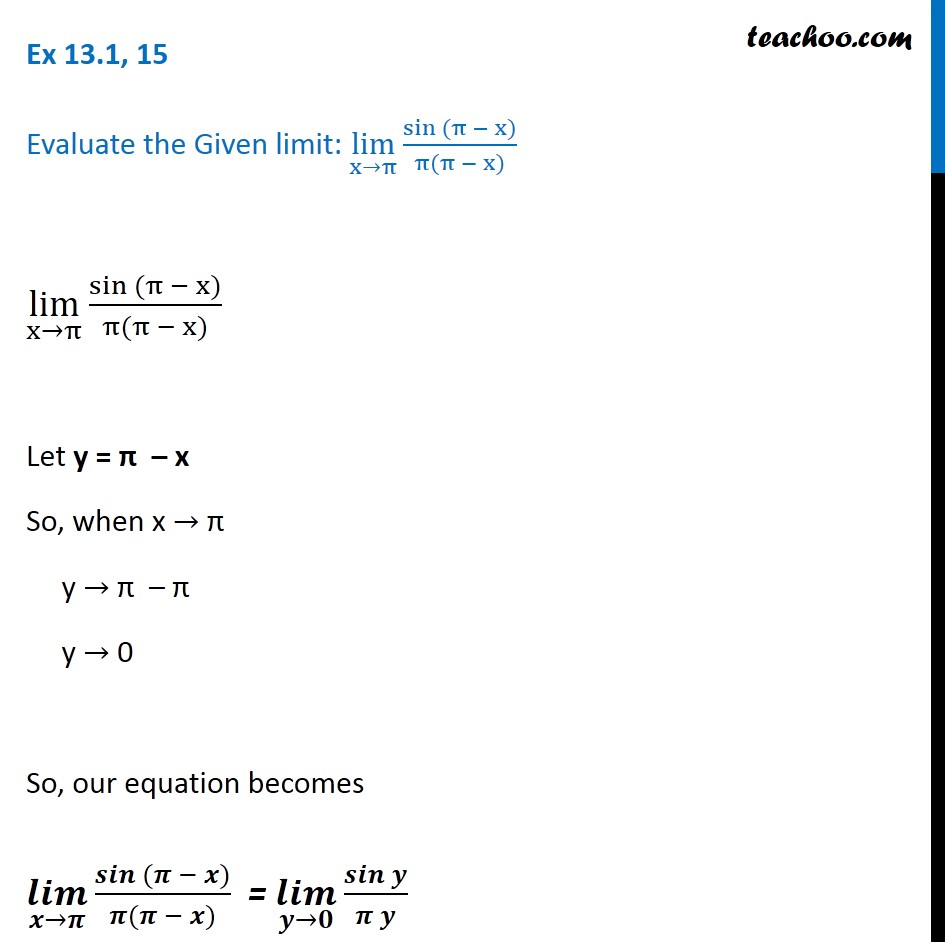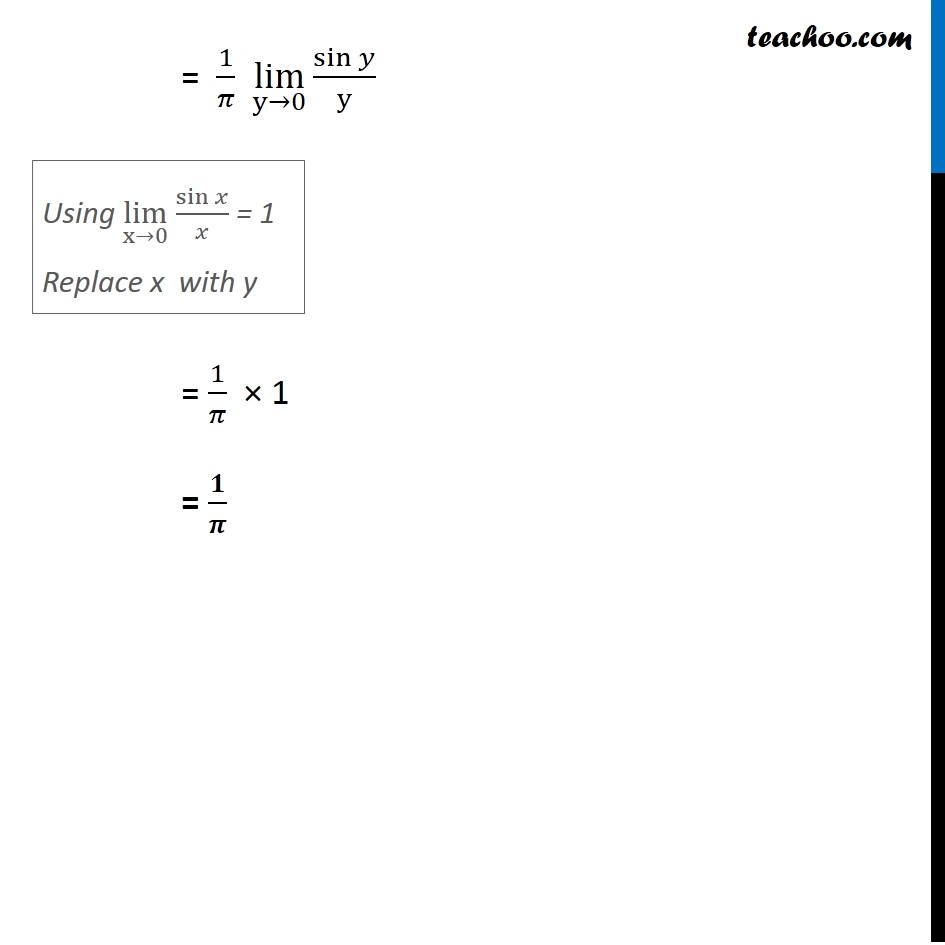Ex 12.1

Chapter 12 Class 11 Limits and Derivatives
Serial order wiseLearn in your speed, with individual attention - Teachoo Maths 1-on-1 Class

### Transcript

Ex 12.1, 15 Evaluate the Given limit: lim┬(x→π) (sin (π − x))/(π(π − x)) lim┬(x→π) (sin (π − x))/(π(π − x)) Let y = π – x So, when x → π y → π – π y → 0 So, our equation becomes (𝒍𝒊𝒎)┬(𝒙→𝝅) (𝒔𝒊𝒏 (𝝅 − 𝒙))/(𝝅(𝝅 − 𝒙)) = (𝒍𝒊𝒎)┬(𝒚→𝟎) (𝒔𝒊𝒏 𝒚)/(𝝅 𝒚) = 1/𝜋 lim┬(y→0) (sin 𝑦)/y = 1/𝜋 × 1 = 𝟏/𝝅 Using lim┬(x→0) sin⁡𝑥/𝑥 = 1 Replace x with y